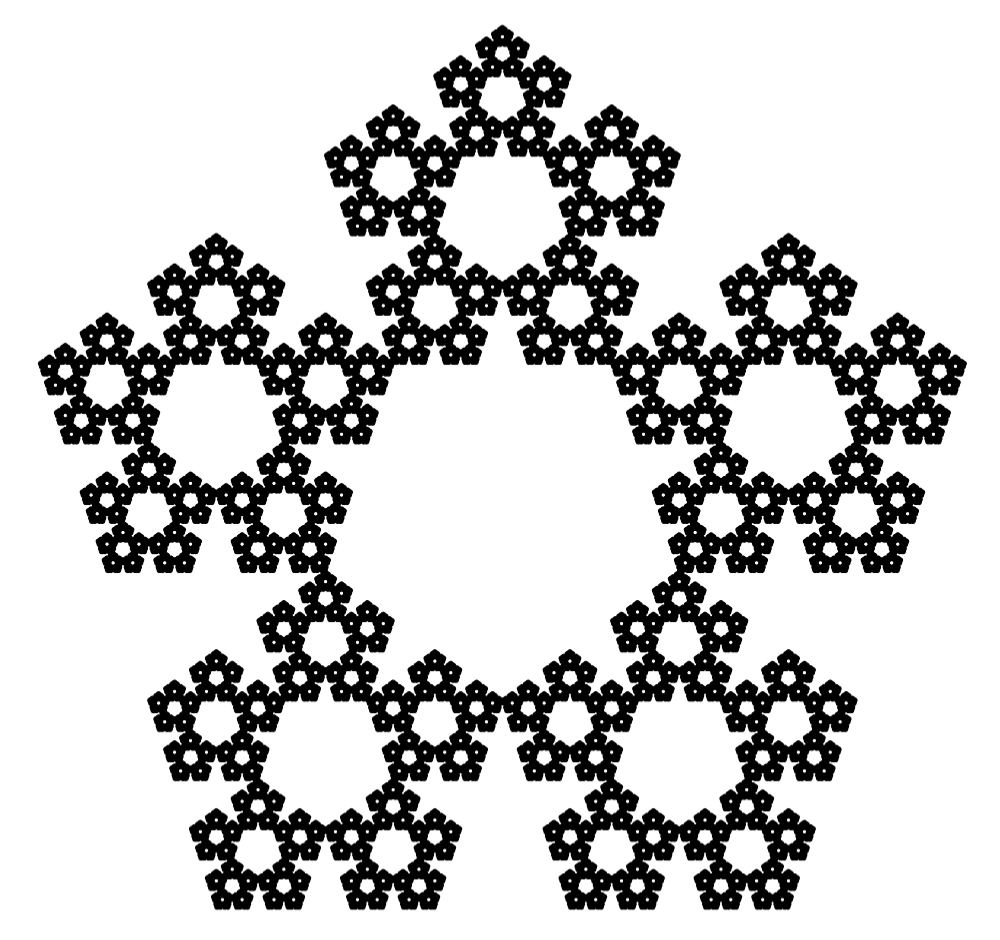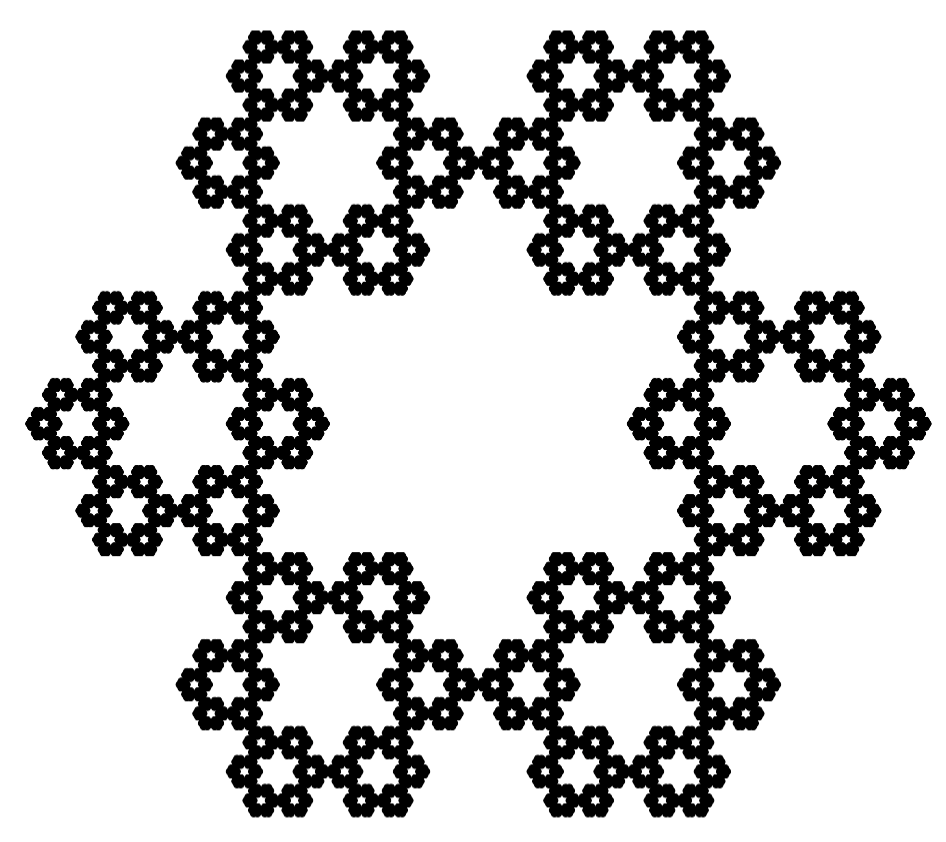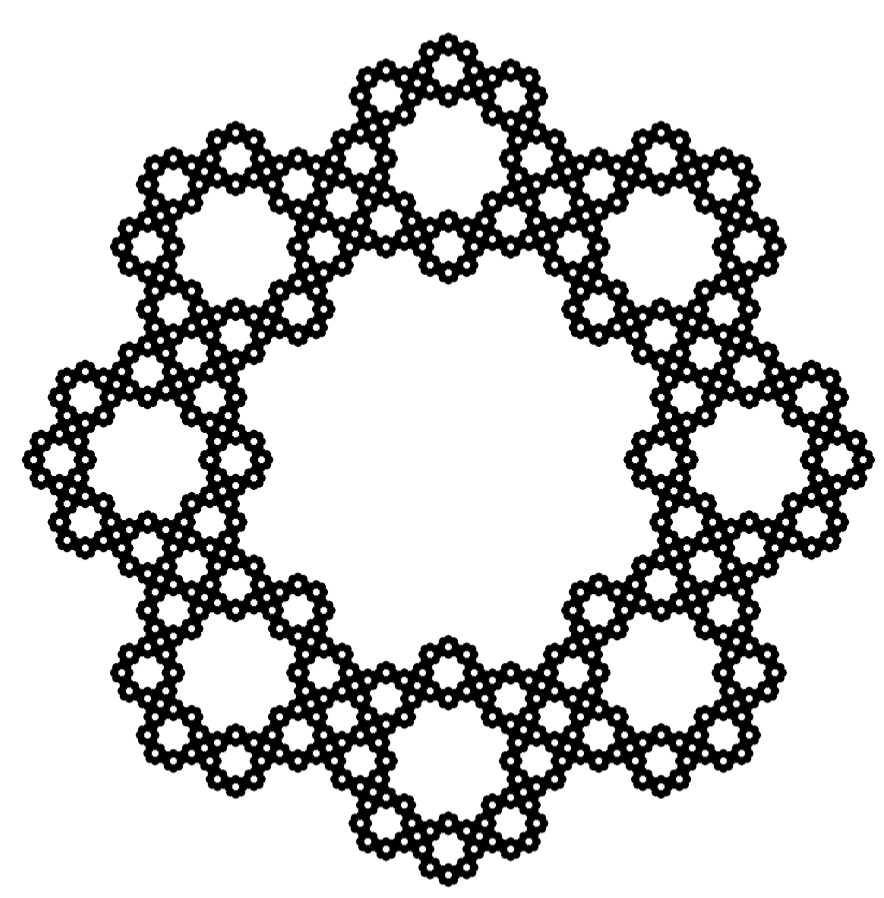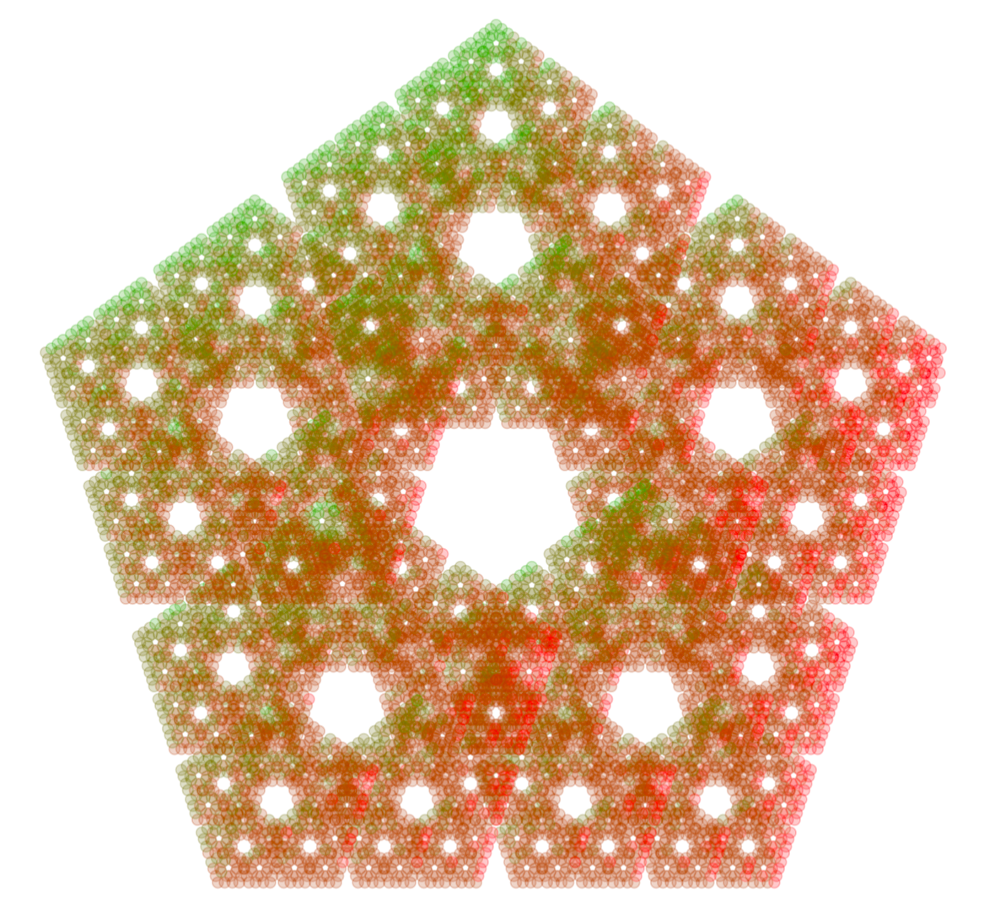## 4.6 Sierpinski polygonsIn the first chapter we showed fractals built from regular polygons with two (Cantor’s dust), three (Sierpiński’s triangle) and four (Sierpiński’s carpet) sides.

It turns out that you can construct a fractal based on any regular polygon. Let $$n$$ denote the number of sides of the polygon. We will construct the fractal by using transformations that either scale or shift the figure.

For the $$n$$-gon, the scaling factor is described by the formula:

$r_n = \frac{1}{{2 \; \left( {1 + \sum\limits_{k = 1}^{\left\lfloor {n/4} \right\rfloor } {\cos \left( {\frac{{2\pi k}}{n}} \right)} } \right)}},$

where $$\left\lfloor {n/4} \right\rfloor$$ is the floor function of $$n/4$$, which is the largest natural number not greater than $$n/4$$.

The scaled figures should be shifted into the sides of the regular polygon, where the shift parameters for $$k$$-th transformation can be derived from the formula:

$(1-r_n) \left[ {\begin{array}{*{20}{c}} { \cos \left( {\frac{{2\pi k}}{n}} \right)} \\ { \sin \left( {\frac{{2\pi k}}{n}} \right)} \\ \end{array}} \right].$

For example, when building a fractal based on a regular pentagon, we should use five transformations. Each scales the figure by:

$r_5 = \left( 2 + 2\cos\left(\frac{2\pi}{5}\right) \right)^{-1} \approx 0.381966$

and shifts by (for k = 1, …, 5):

$(1-r_5)\left[ {\begin{array}{*{20}{c}} { \cos \left( {2\pi k / 5} \right)} \\ { \sin \left( {2\pi k / 5} \right)} \\ \end{array}} \right].$

Examples of fractals for $$n=4; 5; 6; 8$$ are located in the margin.

By experimenting with the scaling factor and with the coloring of individual points, we can get very fancy patterns. Shown in the margin is a Sierpiński pentagon with a scaling factor of 0.4 in which the colors of the points depend on the transformation used.